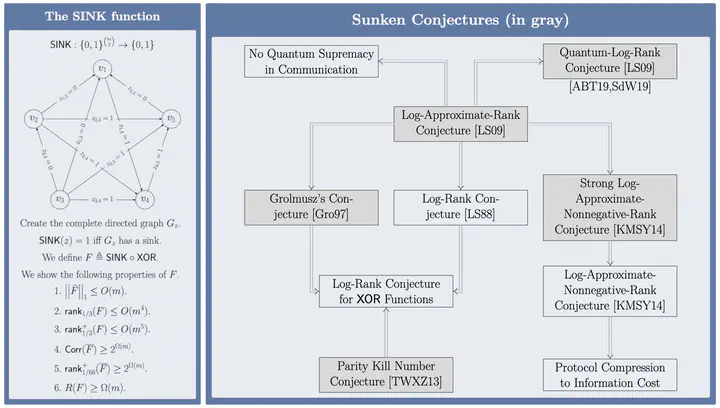# The Log-Approximate-Rank Conjecture Is FalseThe SINK function, some of its properties and the conjectures it refutes.

### Abstract

We construct a simple and total Boolean function $F = f \circ \mathsf{XOR}$ on $2n$ variables that has only $O(\sqrt{n})$ spectral norm, $O(n^2)$ approximate rank and $O(n^{2.5})$ approximate nonnegative rank. (The approximate nonnegative rank has been improved to $O(n^2)$ in this paper by Mande and de Wolf.) We show it has polynomially large randomized bounded-error communication complexity of $\Omega(\sqrt{n})$. This yields the first exponential gap between the logarithm of the approximate rank and randomized communication complexity for total functions. Thus $F$ witnesses a refutation of the Log-Approximate-Rank Conjecture (LARC) which was posed by Lee and Shraibman [LS09] as a very natural analogue for randomized communication of the still unresolved Log-Rank Conjecture for deterministic communication. The best known previous gap for any total function between the two measures is a recent 4th-power separation by Göös, Jayram, Pitassi and Watson [GJPW17].

Additionally, our function $F$ refutes Grolmusz’s Conjecture [Gro97] and a variant of the Log-Approximate-Nonnegative-Rank Conjecture, suggested recently by Kol, Moran, Shpilka and Yehudayoff [KMSY14], both of which are implied by the LARC. The complement of $F$ has exponentially large approximate nonnegative rank. This answers a question of Lee [Lee12] and Kol et al. [KMSY14], showing that approximate nonnegative rank can be exponentially larger than approximate rank. The inner function $f$ also falsifies a conjecture about parity measures of Boolean functions made by Tsang, Wong, Xie and Zhang [TWXZ13]. The latter conjecture implied the Log-Rank Conjecture for $\mathsf{XOR}$ functions.

We are pleased to note that shortly after we published our results two independent groups of researchers, Anshu, Boddu and Touchette [ABT19], and Sinha and de Wolf [SW19], used our function $F$ to prove that the Quantum-Log-Rank Conjecture is also false by showing that $F$ has $\Omega(n^{1/6})$ quantum communication complexity.

Type
Publication
Journal of the ACM##### Suhail Sherif
###### Postdoctoral Researcher

I work in theoretical computer science, with a focus on query and communication complexity.